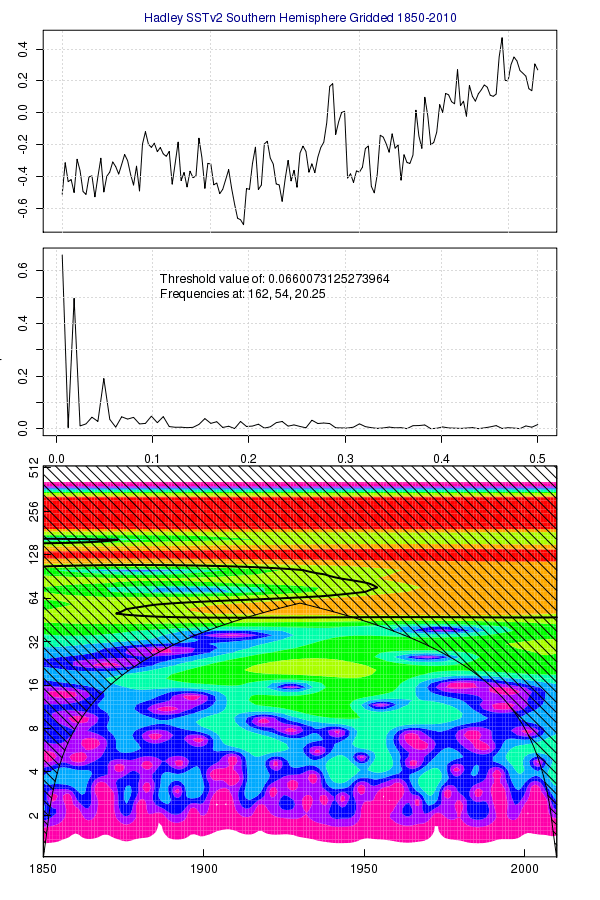Home > HadSST, Natural Variability, Statistics > HadSSTv2: Global, NH, SH Frequency Analysis

## HadSSTv2: Global, NH, SH Frequency Analysis

2011 February 25

This is a mash-up of some of my previous work with Fourier and Morlet analysis extended to a globally gridded data set for sea surface temperatures, the Hadley SSTv2, available as a NetCDF file here. I used this in a ‘trb‘ script last fall. My current attempt is a lot cleaner. I must be learning something. The gridding and masking techniques here are a precursor to more regional studies.

First we read the NetCDF in as a raster ‘brick’, a stack of raster layers available in the R package ‘raster.’ Then extract the ‘sst’ values which are already anomalized to a baseline of 1961-90. If you have an older version of ‘raster’ on your system, run the command ‘update.packages.’ This script needs the newest version.

```# read the nc file

# select sea surface temperatures
SST<-brick(seafile,varname='sst')```

Next, create a Northern Hemisphere and Southern Hemisphere mask to apply to the raster. To generalize the technique, we create a Global mask as well.

```rows = 36 # extract from raster
cols = 72 # extract from raster

rgl <- raster(SST,layer=1)
rgl <- setValues(rgl, c(rep(1,cols*rows)))

rnh <- raster(SST,layer=1)
rnh <- setValues(rnh, c(rep(1,cols*rows/2),rep(NA,cols*rows/2)))

rsh <- raster(SST,layer=1)
rsh <- setValues(rsh,c(rep(NA,cols*rows/2),rep(1,cols*rows/2)))```

I can probably eliminate the following loop (see Mosher), but for now, loop through each raster layer (each layer = one month), and get a latitude weighted global mean.

```# add mask
getRasterLatMean <- function(r) {

rows = 36 # extract from raster
cols = 72 # extract from raster

wcell <- area(r,na.rm=F) # cells
wlat <- extract(wcell,seq(1,((rows-1)*cols+1),cols)) # lat bands

latm <- NULL
latm <- apply(matrix(getValues(r,1,nrows=36),nrow=36),1,mean,na.rm=T)

return(sum(latm*wlat,na.rm=T)/sum(wlat[!is.na(latm)]))
}

###############################################
# Convert Monthly Anomaly to Monthly Mean
# Convert Monthly Mean to Annual Global Mean
###############################################

#-----------------------------------
#-- Get Monthly Mean across Latitudes
#-----------------------------------
glmm <- NULL
nhmm <- NULL
shmm <- NULL
for (j in 1:dim(SST)) {
}```

Now convert the monthly means into annual means.

```#-------------------------------------------
#-- Convert Monthly Mean to Annual
#-------------------------------------------
gl_annmean1 <- apply(t(matrix(glmm,nrow=12)),1,mean,na.rm=T)
nh_annmean1 <- apply(t(matrix(nhmm,nrow=12)),1,mean,na.rm=T)
sh_annmean1 <- apply(t(matrix(shmm,nrow=12)),1,mean,na.rm=T)
```

For comparison sake, I also reversed the order of the above. Instead of ‘monthly anomalies -> global monthly mean -> global annual mean’, I also created a set of ‘monthly anomalies -> annual anomalies -> global annual mean.’

```#-------------------------------------------
#-- Convert to Annual Anomalies
#-------------------------------------------

# vector list of  years
ys <- as.vector(t(matrix(rep(seq(1,200),12),ncol=12)))
# truncated to length of SST
ys <- ys[1:dim(SST)]
# annual means
s <- stackApply(SST,ys,mean)

#-------------------------------------------
#-- Convert to Annual Means
#-------------------------------------------

# for each year in the brick
gl_annmean2 <- NULL
nh_annmean2 <- NULL
sh_annmean2 <- NULL
for (j in 1:dim(s)) {

# calculate mean of each latitude band
r <- raster(s,layer=j)

}```

Comparing these two methods to the ‘published‘ global means, we see that the first method cleaves closest to the official series. (I’ll update with the sum of squared residuals tonight – left those on my pc).After selecting the first method, I created a new plot function to merge some of the Fourier and Morlet plot techniques introduced previously.